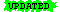#  运算符重载(Part of C++ FAQ Lite, Copyright © 1991-2001, Marshall Cline, cline@parashift.com)

### [13.1] 运算符重载的作用是什么？

class Fred {
public:

// ...
};

#if 0

// 没有运算符重载：
Fred mul(Fred, Fred);

Fred f(Fred a, Fred b, Fred c)
{
// 哈哈，多可笑...
}

#else

// 有运算符重载：
Fred operator+ (Fred, Fred);
Fred operator* (Fred, Fred);

Fred f(Fred a, Fred b, Fred c)
{
return a*b + b*c + c*a;
}

#endif

TopBottomPrevious sectionNext section ]

### [13.2] 运算符重载的好处是什么？

TopBottomPrevious sectionNext section ]

### [13.3] 有什么运算符重载的实例？[Recently changed so it uses the std:: syntax (on 7/00). Click here to go to the next FAQ in the "chain" of recent changes.]

• myString + yourString 可以连接两个 std::string 对象
• myDate++ 可以增加一个 Date 对象
• a * b 可以将两个 Number 对象相乘
• a[i] 可以访问 Array 对象的某个元素
• x = *p 可以反引用一个实际“指向”一个磁盘记录的 "smart pointer" —— 它实际上在磁盘上定位到 p 所指向的记录并返回给x。

TopBottomPrevious sectionNext section ]

### [13.4] 但是运算符重载使得我的类很丑陋；难道它不是应该使我的类更清晰吗？

class Array {
public:
int& operator[] (unsigned i);
// 有些人不喜欢这种语法

// ...
};

inline
int& Array::operator[] (unsigned i)
// 有些人不喜欢这种语法
{

// ...
}

int main()
{
Array a;
a = 4;
// 用户代码应该明显而且易懂...
}

TopBottomPrevious sectionNext section ]

### [13.5] 什么运算符能／不能被重载？

class Array {
public:
int& elem(unsigned i)        { if (i > 99) error(); return data[i]; }
private:
int data;
};

int main()
{
Array a;
a.elem(10) = 42;
a.elem(12) += a.elem(13);
}

class Array {
public:
int& operator[] (unsigned i) { if (i > 99) error(); return data[i]; }
private:
int data;
};

int main()
{
Array a;
a = 42;
a += a;
}

TopBottomPrevious sectionNext section ]

### [13.6] 我能重载 operator== 以便比较两个 char[] 来进行字符串比较吗？[Recently changed so it uses the std:: syntax (on 7/00). Click here to go to the next FAQ in the "chain" of recent changes.]

TopBottomPrevious sectionNext section ]

### [13.7] 我能为“幂”运算创建一个 operator** 吗？

TopBottomPrevious sectionNext section ]

### [13.8] 如何为Matrix（矩阵）类创建下标运算符？[Recently changed so it uses new-style headers and the std:: syntax (on 7/00). Click here to go to the next FAQ in the "chain" of recent changes.]

operator()而不是operator[]

class Matrix {
public:
Matrix(unsigned rows, unsigned cols);
double& operator() (unsigned row, unsigned col);
double  operator() (unsigned row, unsigned col) const;

// ...
~Matrix();
// 析构函数
Matrix(const Matrix& m);
// 拷贝构造函数
Matrix& operator= (const Matrix& m);
// 赋值运算符

// ...
private:
unsigned rows_, cols_;
double* data_;
};

inline
Matrix::Matrix(unsigned rows, unsigned cols)
: rows_ (rows),
cols_ (cols),
data_ (new double[rows * cols])
{
if (rows == 0 || cols == 0)
throw BadIndex("Matrix constructor has 0 size");
}

inline
Matrix::~Matrix()
{
delete[] data_;
}

inline
double& Matrix::operator() (unsigned row, unsigned col)
{
if (row >= rows_ || col >= cols_)
throw BadIndex("Matrix subscript out of bounds");
return data_[cols_*row + col];
}

inline
double Matrix::operator() (unsigned row, unsigned col) const
{
if (row >= rows_ || col >= cols_)
throw BadIndex("const Matrix subscript out of bounds");
return data_[cols_*row + col];
}

int main()
{
Matrix m(10,10);
m(5,8) = 106.15;
std::cout << m(5,8);

// ...
}

TopBottomPrevious sectionNext section ]

### [13.9] 为什么Matrix（矩阵）类的接口不应该象数组的数组？

• operator() 永远不差，是因为用operator()方法实现以行为主的密集矩阵的物理布局非常容易。因此，当从性能观点出发，那样的结构正好是最佳布局时，operator()方法也和[][]方法一样简单（也许operator()方法更容易一点点，但我不想夸大其词）。
• operator() 方法有时更好，是因为当对于给定的应用，有其它比以行为主的密集矩阵更好的布局时，用 operator() 方法比[][]方法实现会容易得多。

TopBottomPrevious sectionNext section ]

### [13.10] 该从外（接口优先）还是从内（数据优先）设计类？[Recently changed so it uses new-style headers and the std:: syntax and reworded references to STL (on 7/00). Click here to go to the next FAQ in the "chain" of recent changes.]

class Stack {
public:

// ...
private:
};

class Node { /*...*/ };

public:

// ...
private:
Node* first_;
};

{
for (LinkedListIterator p = a.begin(); p != a.end(); ++p)
std::cout << *p << '\n';
}

#include <cassert>    // Poor man's exception handling

class Node {

// No public members; this is a "private class"
// 友员类
Node* next_;
int elem_;
};

public:
void operator++ ();
// Go to the next element
int& operator*  ();
// Access the current element
private:
Node* p_;
};

public:
void append(int elem);
// Adds elem after the end
void prepend(int elem);
// Adds elem before the beginning

// ...

// ...
private:
Node* first_;
};

{
return p_ == i.p_;
}

{
return p_ != i.p_;
}

{
assert(p_ != NULL);
// or if (p_==NULL) throw ...
p_ = p_->next_;
}

{
assert(p_ != NULL);
// or if (p_==NULL) throw ...
return p_->elem_;
}

: p_(p)
{ }

{
return first_;
}